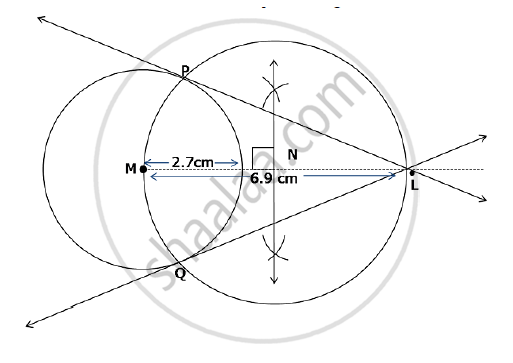# Draw the tangents to the circle from the point L with radius 2.7 cm. Point ‘L’ is at a distance 6.9 cm from the centre ‘M’. - Geometry

Draw the tangents to the circle from the point L with radius 2.7 cm. Point ‘L’ is at a distance 6.9 cm from the centre ‘M’.

#### Solution

Steps of construction:-

Construct a circle with centre M and radius 2.7 cm.

Take point L such that ML = 6.9 cm.

Obtain midpoint N of segment ML.

Draw a circle with centre N and radius NM.

Let P and Q be the points of intersection of these two circles.

Draw lines LP and LQ which are the required tangents.Concept: Construction of Tangents to a Circle
Is there an error in this question or solution?